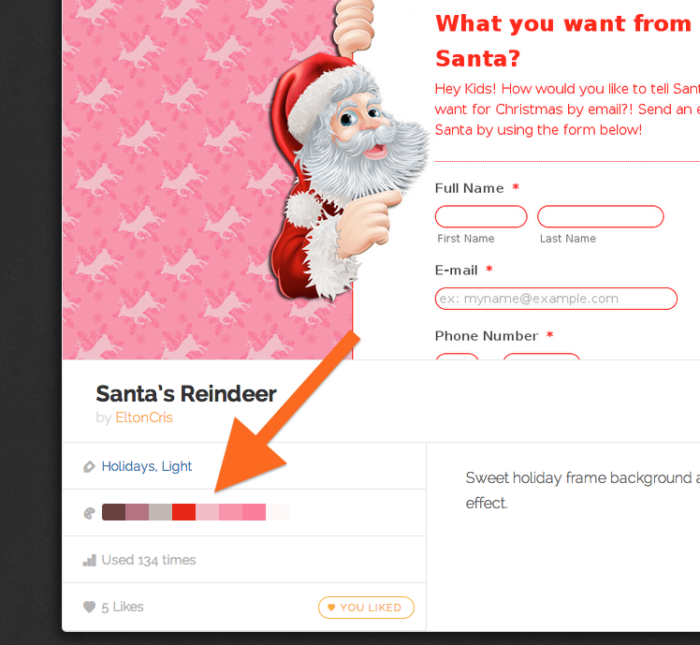# How to Implement Color Search

We have just launched a theme store for our users and we wanted to make it easy to find themes by color. When you are looking for a suitable web form design theme to match your website design, searching based on colors simplifies the process.

In this blog post I will explain how we created color search feature of JotForm Theme Store.You can search for similar themes by clicking on one of the colors on a theme you are looking at. You can see an example by clicking on one of the colors here.

When searching for colors, it is not enough to find exact matches. We need to also find themes with similar colors.

Our first approach was to generate all color values similar to the target color. This way you could search for the themes which contained one of the generated colors. This approach (generating similar color sets) looked like a simple and straightforward solution. But, when we started implementing it, calculating and comparing hundreds of colors turned out to be NOT an effective way to perform a color search.

We then found a better solution. Instead of calculating absolute values of similar colors, we have decided to divide the color spectrum into a certain number of segments, and then use those segment numbers for matching similar colors.

In this method, a segment refers to all similar colors in a specific area of the color spectrum. This means, if two different colors give same result after the segment number calculation, they could be considered as similar colors.

## So, How Do We Calculate a Segment Number?

In the beginning of a color search process we first get the hex value of the target color, and then we convert it to the HSB (Hue Saturation Brightness) color model. Basically, we use HSB color model components to specify a unique number for each segment.

The segment number calculation has 3 steps:

1. Identify the area number according to saturation and brightness values.
2. Identify the layer number according to hue value.
3. Calculate the segment number

We have divided the color spectrum into small pieces. This is a tricky process since the bottom of the color spectrum contains blackish colors while top left area of the spectrum contains colors which are approaching white. As a result of this, we did some adjustment before determining the boundaries of the segments.

In the first step of the calculation, we simply specify the area number according to the color’s saturation and brightness values. It can be a number between 1 and 4 or 145 and 148. If the number is between 1 and 4 it means that the target is a shade of a color. If the area number is between 145 and 148 that means the target color is very close to black, grey or white. That’s how we know a color can be considered in a particular segment number.

Next, we calculate hue partition of the color. In the HSB color model, the hue component can be in an interval between 0 and 360. We have mapped this interval into groups which consists of 10 values. It’s possible to think every group of the hue component as a different layer. So, there would be 36 layers for the hue component. The result of this step finds the number for the corresponding layer.

Imagine that we have a divided color spectrum for every layer of the hue component. Then we give a number to the areas continuously (except black, white and grey) according their layer number. For example, the area number for a color with values (15, 80, 90) is 5. This is exactly what we are doing in the final step of the segment number calculation. The generated segment number of a specific color is a combination of an area number in color spectrum and the hue layer number.

In the color search algorithm that we are using for JotForm Theme Store, we defined 148 different color segments by using this calculation. 144 of these segments are coming from 36 different hue layers that have 4 different areas on their color spectrum. 4 of them (145, 146, 147, 148) are the constant segments for black, dark grey, light grey and white.

## How Do We Match Themes Based on Segment Numbers?

Segment number calculation is needed at two points in our system: saving themes and searching themes.

When someone is saving a new theme, we extract the 8 most commonly used colors and calculate their segment numbers. Completing this heavy work during the saving of the theme allows us to do less work during the search. Converting only search input color to its segment number is sufficient for generating search results.

When a user is searching themes by color, our goal is to return all similar colors as well, so we convert the RGB color model to the HSB color model, calculate its segment number and then return themes which contain the same segment number.

When we decided we needed a color search for the JotForm Theme Store, we had no idea how much fun we were in for. We are excited to know that our users can now browse stunning form themes by color thanks to the simple solution we found.Be the first to comment.
•